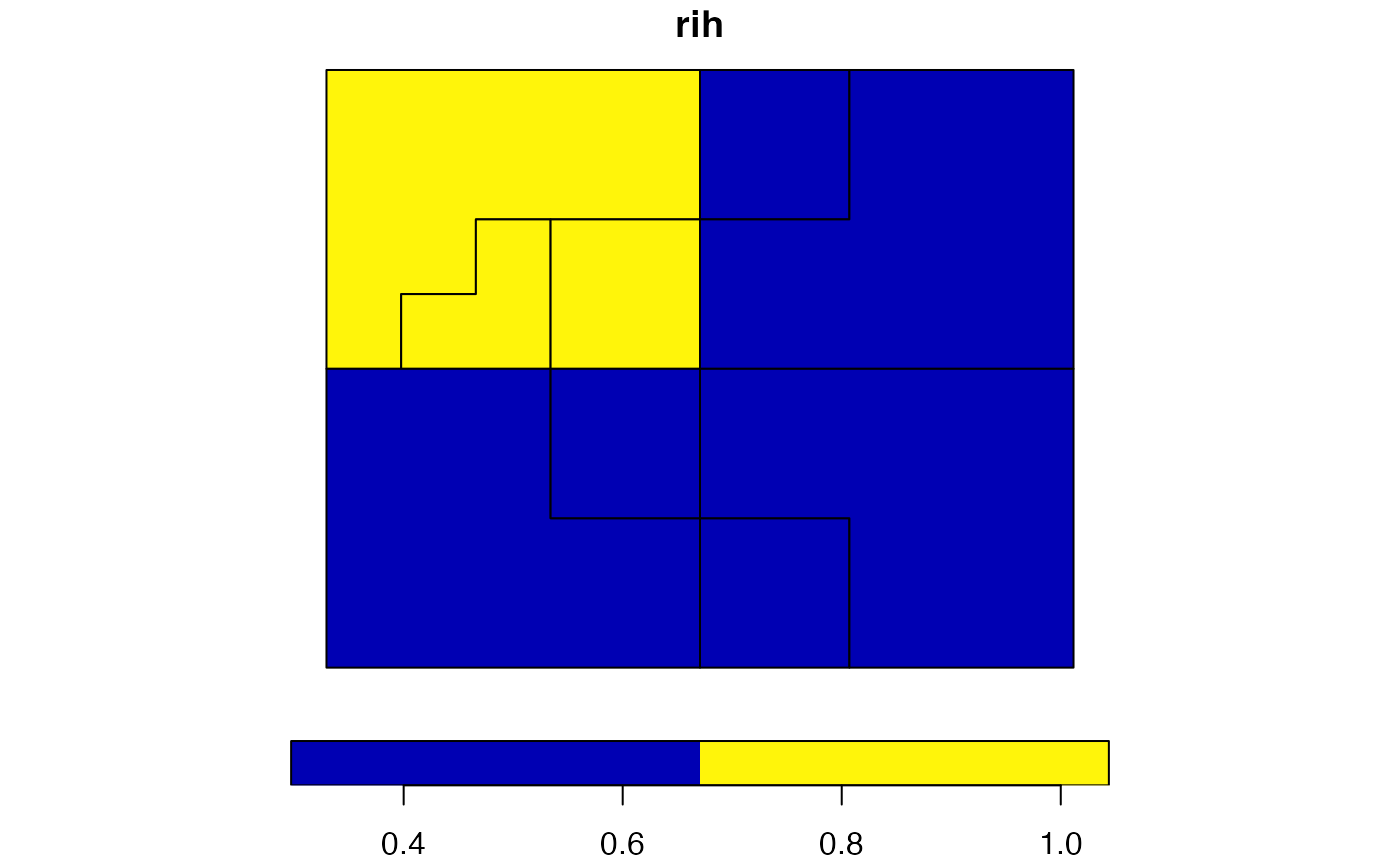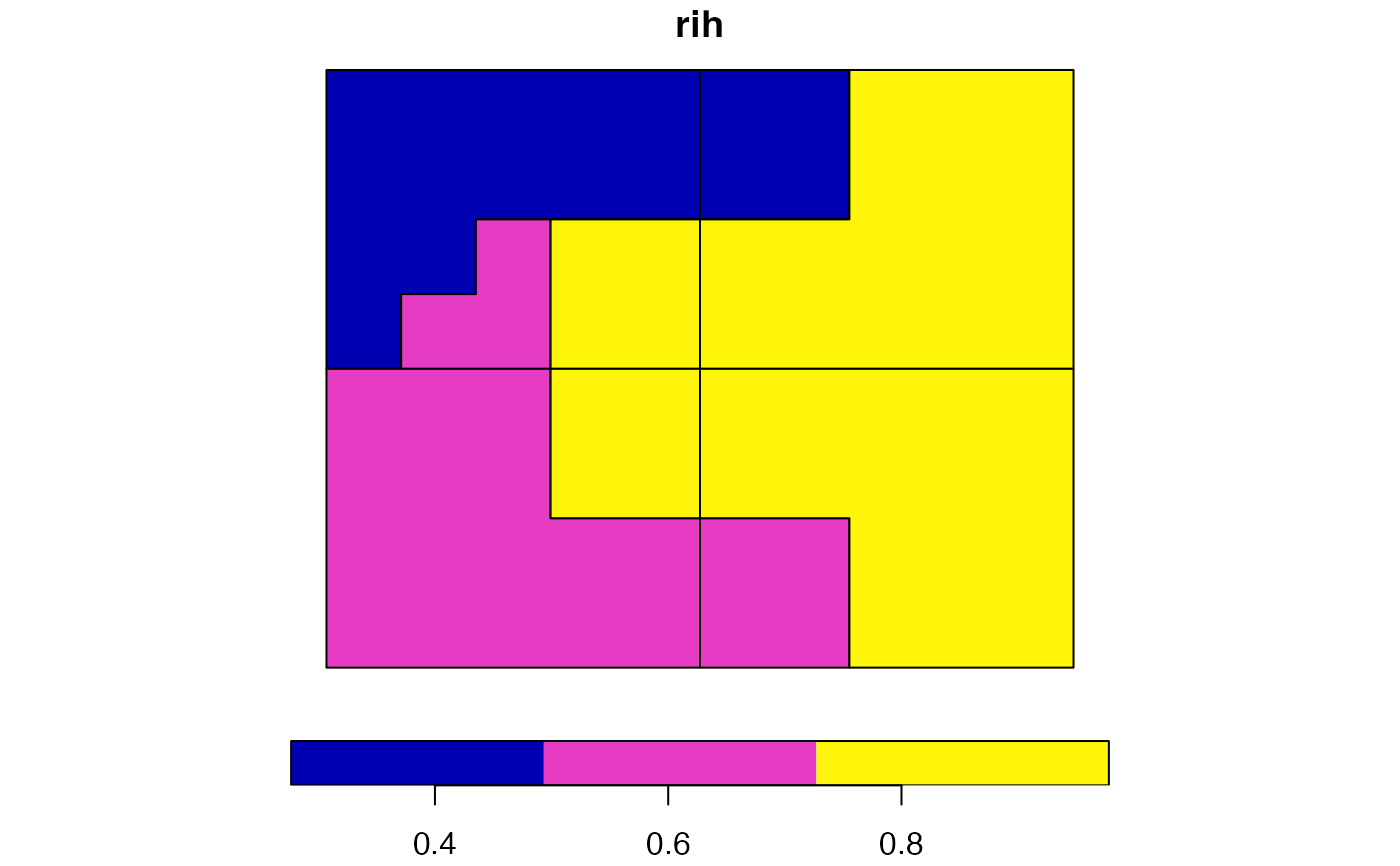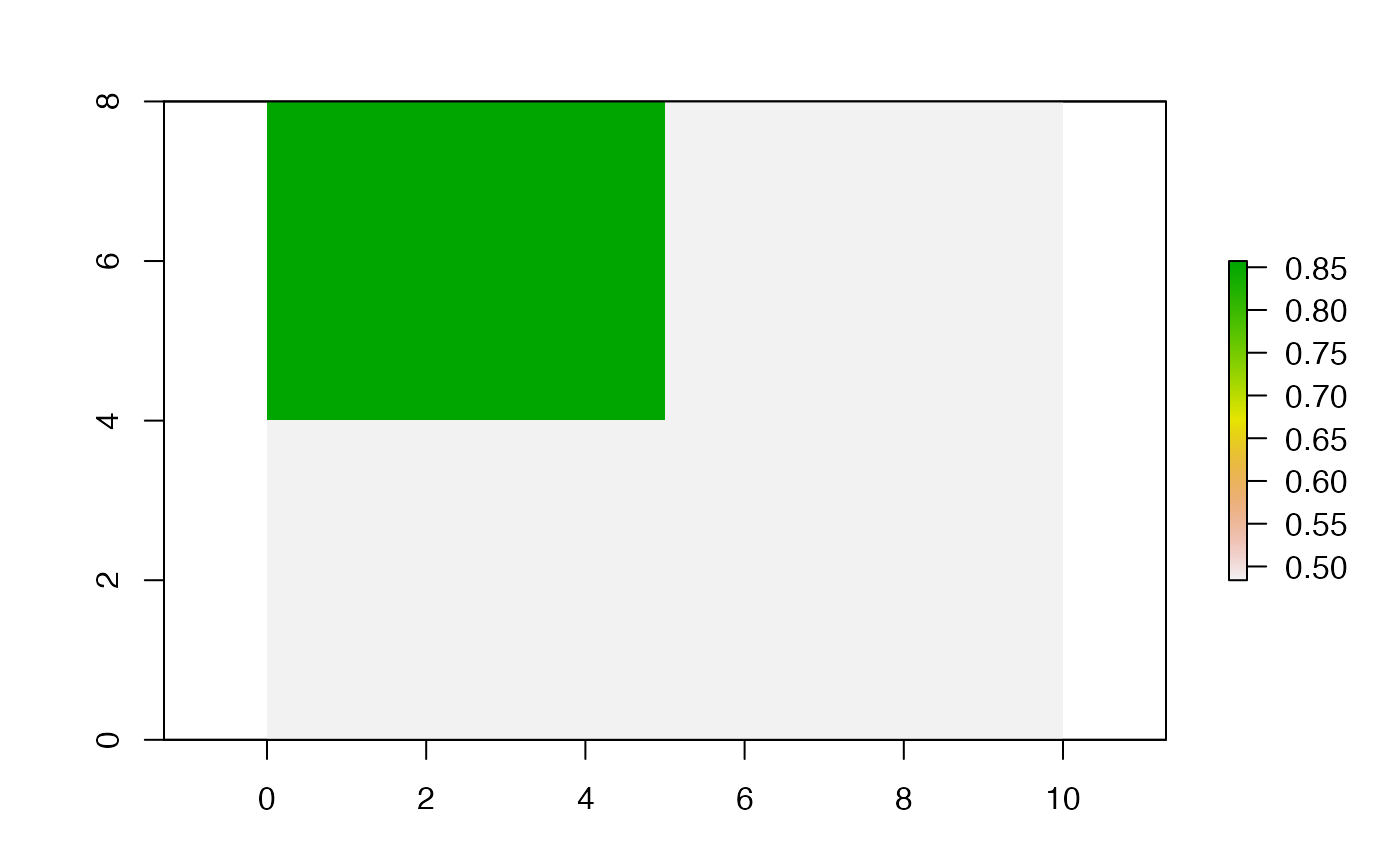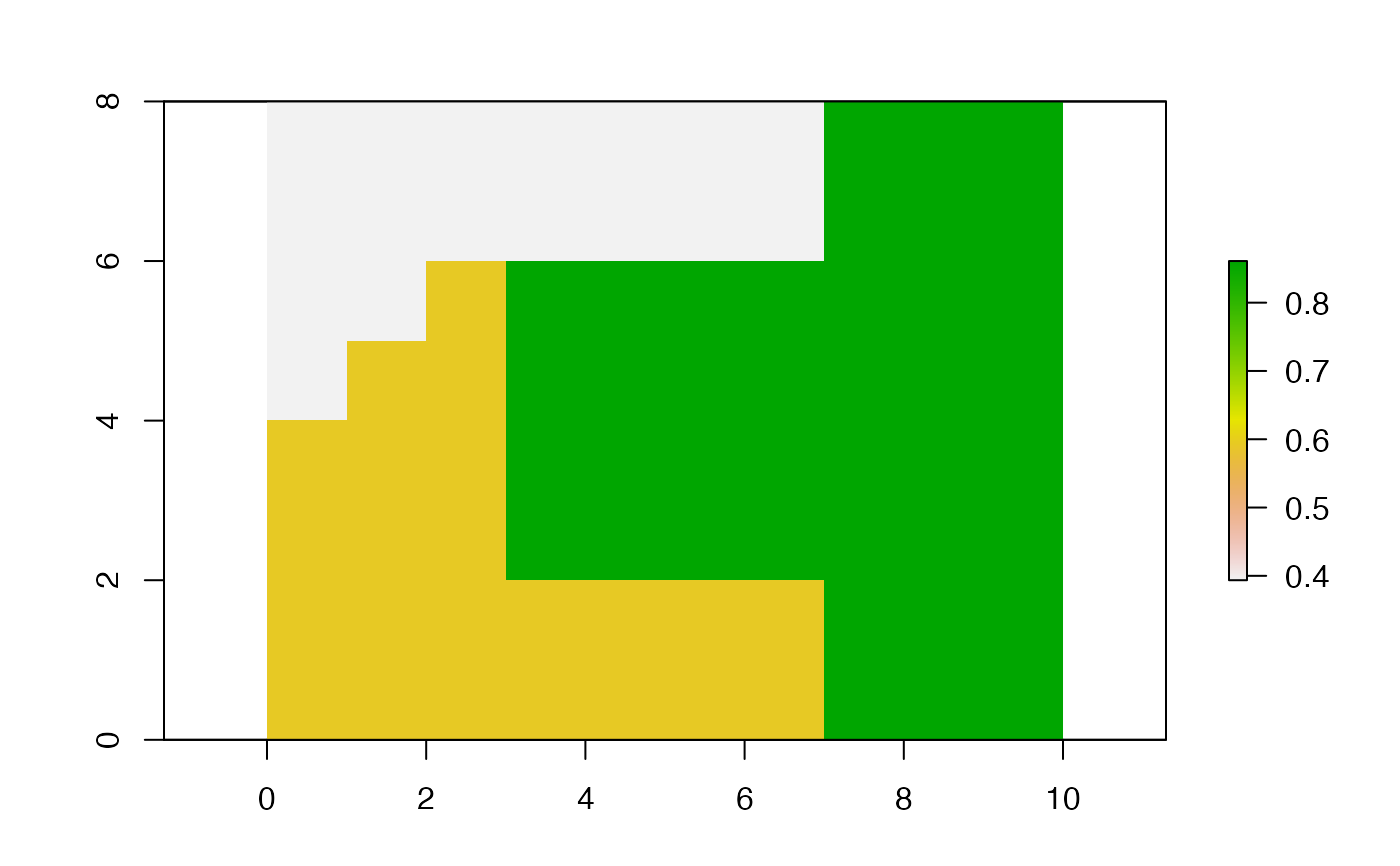It calculates a degree of spatial association between regionalizations using an information-theoretical measure called the V-measure

vmeasure_calc(x, y, x_name, y_name, B = 1, precision = NULL)

# S3 method for sf
vmeasure_calc(x, y, x_name, y_name, B = 1, precision = NULL)

# S3 method for stars
vmeasure_calc(x, y, x_name = NULL, y_name = NULL, B = 1, precision = NULL)

# S3 method for SpatRaster
vmeasure_calc(x, y, x_name = NULL, y_name = NULL, B = 1, precision = NULL)

# S3 method for RasterLayer
vmeasure_calc(x, y, x_name = NULL, y_name = NULL, B = 1, precision = NULL)

## Arguments

x

An object of class sf with a POLYGON or MULTIPOLYGON geometry type or a spatial raster object of class RasterLayer, SpatRaster, or stars.

y

An object of class sf with a POLYGON or MULTIPOLYGON geometry type or a spatial raster object of class RasterLayer, SpatRaster, or stars.

x_name

A name of the column with regions/clusters names.

y_name

A name of the column with regions/clusters names.

B

A numeric value. If B > 1 then completeness is weighted more strongly than homogeneity, and if B < 1 then homogeneity is weighted more strongly than completeness. By default this value is 1.

precision

numeric, or object of class units with distance units (but see details); see st_as_binary for how to do this.

## Value

A list with five elements:

• "map1" - the sf object containing the first preprocessed map used for calculation of GOF with two attributes - map1 (name of the category) and rih (region inhomogeneity)

• "map2" - the sf object containing the second preprocessed map used for calculation of GOF with two attributes - map1 (name of the category) and rih (region inhomogeneity)

• "v_measure"

• "homogeneity"

• "completeness"

## Examples

library(sf)
data("regions1")
data("regions2")
vm = vmeasure_calc(x = regions1, y = regions2, x_name = z, y_name = z)
vm
#> The SABRE results:
#>
#>  V-measure: 0.36
#>  Homogeneity: 0.32
#>  Completeness: 0.42
#>
#>  The spatial objects can be retrieved with:
#>  $map1 - the first map #>$map2 - the second map

plot(vm$map1["rih"])plot(vm$map2["rih"])library(raster)
data("partitions1")
data("partitions2")
vm2 = vmeasure_calc(x = partitions1, y = partitions2)
vm2
#> The SABRE results:
#>
#>  V-measure: 0.36
#>  Homogeneity: 0.32
#>  Completeness: 0.42
#>
#>  The spatial objects can be retrieved with:
#>  $map1 - the first map #>$map2 - the second map

plot(vm2$map1[["rih"]])plot(vm2$map2[["rih"]])